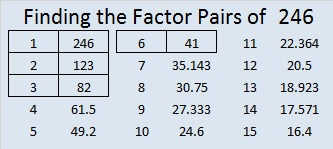# 246 and Level 1

• 246 is a composite number.
• Prime factorization: 246 = 2 x 3 x 41
• The exponents in the prime factorization are 1, 1, and 1. Adding one to each and multiplying we get (1 + 1)(1 + 1)(1 + 1) = 2 x 2 x 2 = 8. Therefore 246 has 8 factors.
• Factors of 246: 1, 2, 3, 6, 41, 82, 123, 246
• Factor pairs: 246 = 1 x 246, 2 x 123, 3 x 82, or 6 x 41
• 246 has no square factors so its square root cannot be simplified. √246 ≈ 15.684Print the puzzles or type the factors on this excel file: 10 Factors 2014-09-29This site uses Akismet to reduce spam. Learn how your comment data is processed.